In an electrical cable, there is a single wire of radius 9 mm of copper. Its resistance is 5 Ω. The cable is replaced by 6 different insulated copper wires, the radius of each wire is 3 mm. Now the total resistance of the cable will be :

(1) 7.5 Ω

(2) 45 Ω

(3) 90 Ω

(4) 270 Ω

Concept Questions :-

Combination of resistors
High Yielding Test Series + Question Bank - NEET 2020

Difficulty Level:

Two uniform wires A and B are of the same metal and have equal masses. The radius of wire A is twice that of wire B. The total resistance of A and B when connected in parallel is :

(1) 4 Ω when the resistance of wire A is 4.25 Ω

(2) 5 Ω when the resistance of wire A is 4.25 Ω

(3) 4 Ω when the resistance of wire B is 4.25 Ω

(4) 4 Ω when the resistance of wire B is 4.25 Ω

Concept Questions :-

Derivation of Ohm's law
High Yielding Test Series + Question Bank - NEET 2020

Difficulty Level:

Twelve wires of equal length and same cross-section are connected in the form of a cube. If the resistance of each of the wires is R, then the effective resistance between the two diagonal ends would be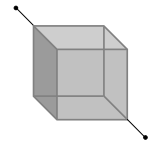(1) 2R

(2) 12R

(3) $\frac{5}{6}R$

(4) 8R

Concept Questions :-

Combination of resistors
High Yielding Test Series + Question Bank - NEET 2020

Difficulty Level:

You are given several identical resistances each of value R = 10 Ω and each capable of carrying maximum current of 1 ampere. It is required to make a suitable combination of these resistances to produce a resistance of 5 Ω which can carry a current of 4 amperes. The minimum number of resistances of the type R that will be required for this job

(1) 4

(2) 10

(3) 8

(4) 20

Concept Questions :-

Combination of resistors
High Yielding Test Series + Question Bank - NEET 2020

Difficulty Level:

The resistance of a wire is 10–6 Ω per metre. It is bend in the form of a circle of diameter 2 m. A wire of the same material is connected across its diameter. The total resistance across its diameter AB will be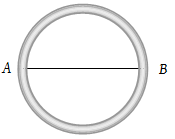(1) $\frac{4}{3}\pi ×{10}^{-6}\Omega$

(2) $\frac{2}{3}\pi ×{10}^{-6}\Omega$

(3) $0.88×{10}^{-6}\Omega$

(4) $14\pi ×{10}^{-6}\Omega$

Concept Questions :-

Combination of resistors
High Yielding Test Series + Question Bank - NEET 2020

Difficulty Level:

In the figure shown, the capacity of the condenser C is $2\text{\hspace{0.17em}}\mu F$. The current in 2 Ω resistor is :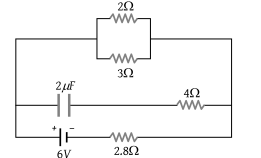(1) 9 A

(2) 0.9 A

(3) $\frac{1}{9}A$

(4) $\frac{1}{0.9}A$

Concept Questions :-

Kirchoff's voltage law
High Yielding Test Series + Question Bank - NEET 2020

Difficulty Level:

When the key K is pressed at time t = 0, which of the following statements about the current I in the resistor AB of the given circuit is true?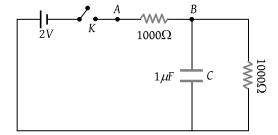(1) I = 2 mA at all t

(2) I oscillate between 1 mA and 2mA

(3) I = 1 mA at all t

(4) At t = 0 , I = 2 mA and with time it goes to 1 mA

Concept Questions :-

Kirchoff's voltage law
High Yielding Test Series + Question Bank - NEET 2020

Difficulty Level:

A torch bulb rated as 4.5 W, 1.5 V is connected as shown in the figure. The e.m.f. of the cell needed to make the bulb glow at full intensity is :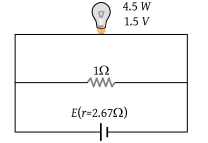(1) 4.5 V

(2) 1.5 V

(3) 2.67 V

(4) 13.5 V

Concept Questions :-

Heating effect of current
High Yielding Test Series + Question Bank - NEET 2020

Difficulty Level:

In the circuit shown in the figure, the current through :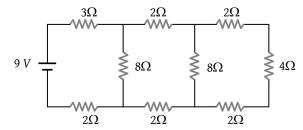1. The 3Ω resistor is 0.50A

2. The 3Ω resistor is 0.25 A

3. The 4Ω resistor is 0.50A

4. The 4Ω resistor is 0.25 A

Concept Questions :-

Combination of resistors
High Yielding Test Series + Question Bank - NEET 2020

Difficulty Level:

There are three resistance coils of equal resistance. The maximum number of resistances you can obtain by connecting them in any manner you choose, being free to use any number of the coils in any way is :

(1) 3

(2) 4

(3) 6

(4) 5

Concept Questions :-

Combination of resistors